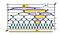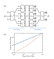# Quantum Convolution Neural Networks

Convolution Neural networks are a breed of neural network-based representation learning architecture that used convolution operation to downsample a large N-dimensional feature map while keeping the important information intact in the low dimensional representations. I will not get into the details of what are CNNs, how do they work. What is convolution operation, what is max-pooling, average pooling, or what is global pooling? I expect the viewer of this article have a fair understanding of these concepts and part from that have a fair understanding of quantum arithmetics and quantum gates.

# Cluster -State Quantum Computation

We will be using the cluster state as an input to the Quantum Convolution Neural Network so let’s get an idea or develop an intuition behind it’s concept.

• We take sequence of single-qubit measurements applied to a fixed quantum state known as a cluster state.
• As an overview of the quantum circuit model for computation includes the following steps:
1) Allowing the input state to be any tensor product of single-qubit states, |ψ1i⊗|ψ2i⊗. . .⊗|ψni
2) Allowing measurements with respect to any orthonormal single-qubit basis, since this is equivalent to applying a single-qubit unitary operation followed by a computational basis measurement.
3) Allowing measurements and feedforward of the measurement results during the computation, so later actions (e.g., quantum gates) may depend on the results of earlier measurement outcomes.

## The cluster state model

• A cluster-state computation begins with the preparation of a special entangled many qubit quantum states, known as a cluster state, followed by an adaptive sequence of single-qubit measurements, which process the cluster, and finally read-out of the computation’s result from the remaining qubits.
• The idea is that to any graph G on n vertices we can define an associated n-qubit cluster state, by first associating to each vertex a corresponding qubit, and then applying a graph-dependent preparation procedure to the qubits, as described below.
• Labels indicate qubits on which processing measurements occur, while unlabeled qubits are those which remain as the output of the computation when the processing measurements are complete. Note that the qubits are labeled by a positive integer n and a single-qubit unitary, which we refer to generically as U; here U = HZ±αj , HZ±βj . The n label indicates the time-ordering of the processing measurements, with qubits having the same label capable of being measured in either order or simultaneously. The time order is important because it determines which measurement results can be fed-forward to control later measurement bases. The U label indicates the basis in which the qubit is measured, denoting a rotation by the unitary U, followed by a computational basis measurement. Equivalently, a single-qubit measurement on the basis {U † |0i, U† |1i} is performed.
• Conversely, it is straightforward to see that any cluster-state computation may be efficiently simulated in the quantum circuit model, and thus the two models are computationally equivalent.

# Overview(QCNN)

• A convolution layer applies a single quasi-local unitary (Ui) in a translational invariant manner for finite depth. For pooling, a fraction of qubits are measured, and their outcomes determine unitary rotations (Vj ) applied to nearby qubits. Hence, nonlinearities in QCNN arise from reducing the number of degrees of freedom. Convolution and pooling layers are performed until the system size is sufficiently small; then, a fully connected layer is applied as a unitary F on the remaining qubits. Finally, the outcome of the circuit is obtained by measuring a fixed number of output qubits.
• QCNNs circuits are related to two well-known concepts in quantum information theory. The multiscale entanglement renormalization ansatz(MERA) and Quantum error correction.A MERA M inherits the causal structure of quantum circuit C: the causal cone C [s] for site s has bounded width. When reversing the arrow of time θ, M implements entanglement renormalization transformations.

# MERA and QEC

• QCNN is specifically designed to contain the MERA representation of the 1D cluster state (|ψ0i) — the ground state of H with h1 = h2 = 0 — such that it becomes a stable fixed point. When |ψ0i is fed as input, each convolution-pooling unit produces the same state |ψ0i with reduced system size in the unmeasured qubits, while yielding deterministic outcomes (X = 1) in the measured qubits. The fully connected layer measures the SOP for |ψ0>.
• When an input wavefunction is perturbed away from |ψ0>, QCNN corrects such “errors.” For example, if a single X error occurs, the first pooling layer identifies its location, and controlled unitary operations correct the error propagated through the circuit.
• Similarly, if an initial state has multiple, sufficiently separated errors (possibly incoherent superpositions), the error density after several iterations of convolution and pooling layers will be significantly smaller. If the input state converges to the fixed point, our QCNN classifies it into the SPT phase with high fidelity.The schematic diagram for using QCNNs to optimize QEC. The inverse QCNN encodes a single logical qubit |ψli into 9 physical qubits, which undergo noise N.QCNN then decodes these to obtain the logical state ρ.aim is to maximize hψl| ρ |ψli.Logical error rate of Shor code (blue) versus a learned QEC code (orange) in a correlated error model. The input error rate is defined as the sum of all probabilities pµ and pxx.

This is a very high view of the QCNNs, it is kind of heavy stuff and a lot of fundamental concepts are needed to be studied before getting to know it’s theory overall. Some notes I could gather from the papers around it.

# References :

Minimalist Stochastic Bayesian

## More from Amit Singh Bhatti

Minimalist Stochastic Bayesian